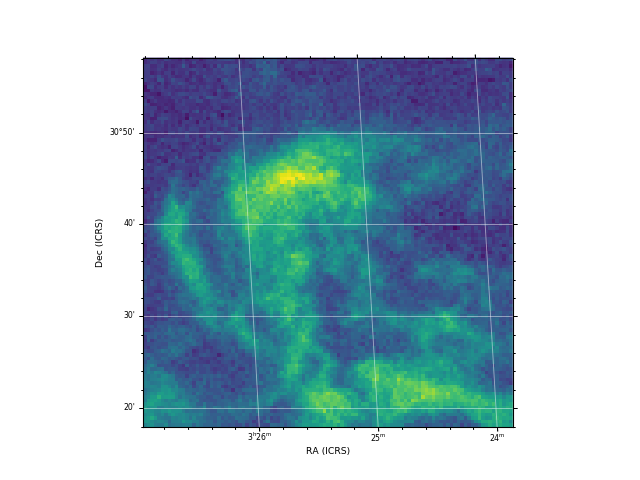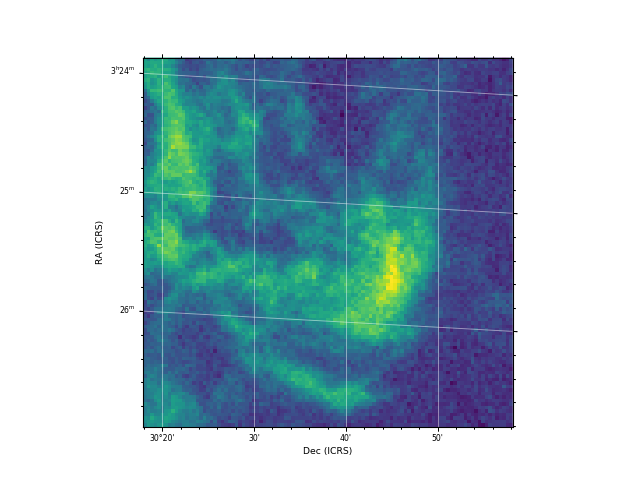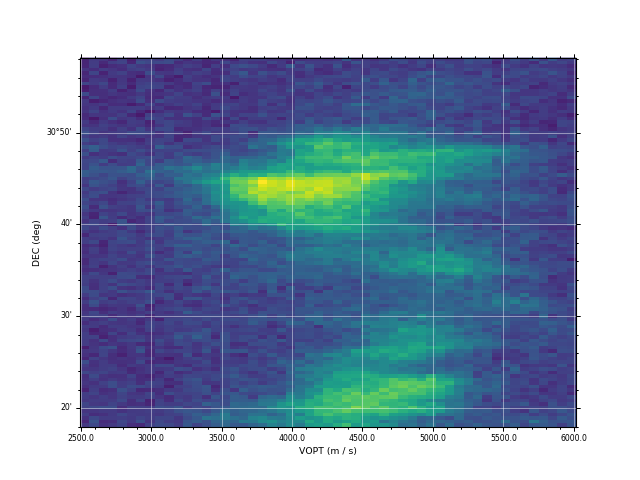# Slicing multi-dimensional data cubes¶

## How to slice data cubes¶

APLpy supports extracting a slice from n-dimensional FITS cubes, and re-ordering dimensions. The two key arguments to `FITSFigure` to control this are `dimensions` and `slices`. These arguments can also be passed to `show_contour()`.

The `dimensions` argument is used to specify which dimensions should be used for the x- and y-axis respectively (zero based). The default values are ```[0, 1]``` which means that the x-axis should use the first dimension in the FITS cube, and the y-axis should use the second dimension. For a 2-dimensional FITS file, this means that one can use `[1, 0]` to flip the axes. For a FITS cube with R.A., Declination, and Velocity, `[0, 2]` would make a R.A.-Velocity plot.

The `slices` argument gives the pixels slice to extract from the remaining dimensions, skipping the dimensions used, so `slices` should be a list with length n-2 where n is the number of dimensions in the FITS file. For example, if one has a FITS file with R.A., Declination, Velocity, and Time (in that order), then:

• `dimensions=[0, 1]` means the plot will be an R.A-Declination plot, and `slices=[33, 56]` means that pixel slices 33 and 56 will be used in Velocity and Time respectively (in this case, `dimensions` does not need to be specified since it defaults to `[0, 1]`)
• `dimensions=[0, 2]` means the plot will be an R.A-Velocity plot, and `slices=[22, 56]` means that pixel slices 22 and 56 will be used in Declination and Time respectively.
• `dimensions=[3, 2]` means the plot will be a Time-Velocity plot, and `slices=[10, 22]` means that pixel slices 10 and 22 will be used in R.A and Declination respectively.

See Arbitrary coordinate systems for information on formatting the labels when non-longitude/latitude coordinates are used.

## Aspect ratio¶

When plotting images in sky coordinates, APLpy makes pixel square by default, but it is possible to change this. When calling `show_grayscale()` or `show_colorscale()`, simply add `aspect='auto'` which will override the `aspect='equal'` default. The `aspect='auto'` is demonstrated below.

## Example¶

The following examples demonstrates this functionality in use

```from astropy.utils.data import get_pkg_data_filename
co_cube = get_pkg_data_filename('l1448/l1448_13co.fits')

import aplpy

f = aplpy.FITSFigure(co_cube, slices=, figsize=(8, 6))
f.show_colorscale()
f.tick_labels.set_font(size='x-small')
f.axis_labels.set_font(size='small')
``````from astropy.utils.data import get_pkg_data_filename
co_cube = get_pkg_data_filename('l1448/l1448_13co.fits')

import aplpy

f = aplpy.FITSFigure(co_cube, slices=,
dimensions=[1, 0], figsize=(8, 6))
f.show_colorscale()
f.tick_labels.set_font(size='x-small')
f.axis_labels.set_font(size='small')
``````from astropy.utils.data import get_pkg_data_filename
co_cube = get_pkg_data_filename('l1448/l1448_13co.fits')

import aplpy

f = aplpy.FITSFigure(co_cube, dimensions=[2, 1],
slices=, figsize=(8, 6))
f.show_colorscale(aspect='auto')